# 前言

DirectX11 With Windows SDK完整目录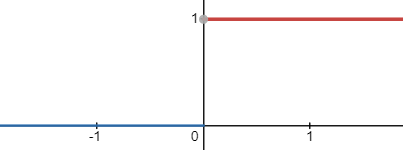$f(d_r)=P(d_o\geq d_r)$

$\mu=E(d_o)\\ \sigma^2=E(d_o^2)-E(d_o)^2$

$E(x)=\int xp(x)dx\\ E(x^2)=\int x^2p(x)dx$

$E(d_o)\approx\sum w_i d_i\\ E(d_o^2)\approx\sum w_i d_i^2$

$P(d_o\geq d_r)\leq p_{max}(d_r)\equiv \frac{\sigma^2}{\sigma^2+(\mu-d_r)^2}$

$\sigma^2=0, \mu=d_r$时，上式未定义，为此可以在分子分母同时加上一个极小量$\epsilon$，或者是$\sigma^2<\epsilon$时直接让$\sigma^2:=\epsilon$。此时没有遮蔽的话值为1； 产生遮蔽的话值接近0。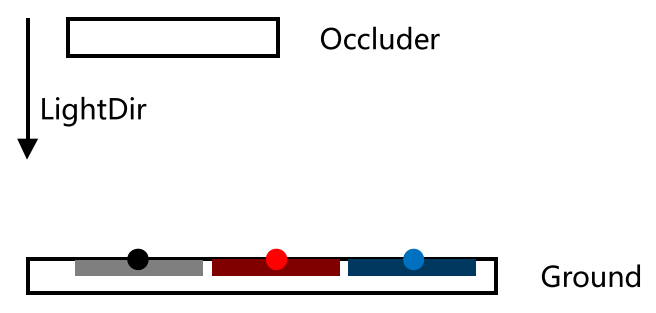float ChebyshevUpperBound(float2 moments,
float minVariance,
float lightBleedingReduction)
{
float variance = moments.y - (moments.x * moments.x);
variance = max(variance, minVariance); // 防止0除

float d = receiverDepth - moments.x;
float p_max = variance / (variance + d * d);

// 单边切比雪夫
return (receiverDepth <= moments.x ? 1.0f : p_max);
}


## 对VSM滤波

• 使用MSAA记录更多深度
• 使用盒型滤波或高斯滤波处理方差阴影贴图
• 使用mipmap

// Shadow.hlsl

float2 texCoord : TEXCOORD) : SV_Target
{
uint2 coords = uint2(posH.xy);

float2 depth;
depth.y = depth.x * depth.x;
return depth;
}


// Shadow.hlsl
#ifndef BLUR_KERNEL_SIZE
#define BLUR_KERNEL_SIZE 3
#endif

static const int BLUR_KERNEL_BEGIN = BLUR_KERNEL_SIZE / -2;
static const int BLUR_KERNEL_END = BLUR_KERNEL_SIZE / 2 + 1;
static const float FLOAT_BLUR_KERNEL_SIZE = (float)BLUR_KERNEL_SIZE;

Texture2D g_TextureShadow : register(t1);                      // 用于模糊
SamplerState g_SamplerPointClamp : register(s0);

float2 VSMHorizontialBlurPS(float4 posH : SV_Position,
float2 texcoord : TEXCOORD) : SV_Target
{
float2 depths = 0.0f;
[unroll]
for (int x = BLUR_KERNEL_BEGIN; x < BLUR_KERNEL_END; ++x)
{
depths += g_TextureShadow.Sample(g_SamplerPointClamp, texcoord, int2(x, 0));
}
depths /= FLOAT_BLUR_KERNEL_SIZE;
return depths;
}

float2 VSMVerticalBlurPS(float4 posH : SV_Position,
float2 texcoord : TEXCOORD) : SV_Target
{
float2 depths = 0.0f;
[unroll]
for (int y = BLUR_KERNEL_BEGIN; y < BLUR_KERNEL_END; ++y)
{
depths += g_TextureShadow.Sample(g_SamplerPointClamp, texcoord, int2(0, y));
}
depths /= FLOAT_BLUR_KERNEL_SIZE;
return depths;
}


## 漏光(Light Bleeding)

VSM最大的问题在于漏光现象，见下图（不得不说这漏光是真的严重）。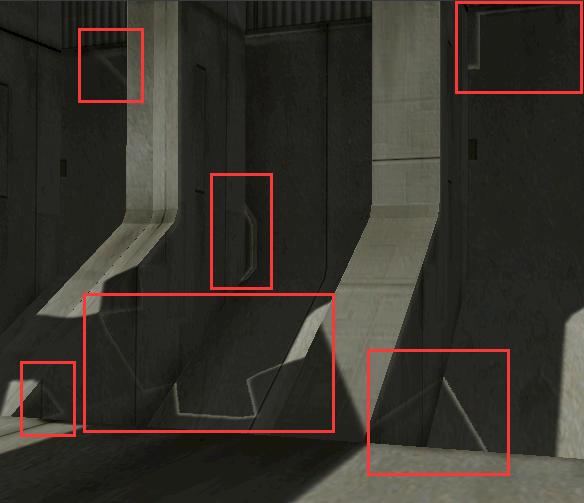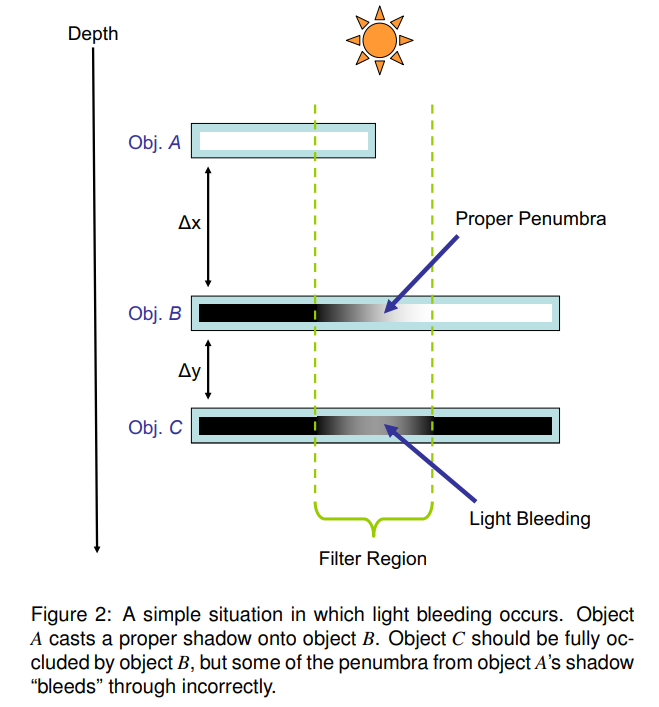$M_1=\frac{a+b}{2}\\ M_2=\frac{a^2+b^2}{2}$

$\mu=\frac{a+b}{2}\\ \sigma^2=M_2^2-(M_1)^2=\frac{(a-b)^2}{4}$

\begin{aligned} p(\Delta{y})&=\frac{\frac{1}{4}\Delta{x^2}}{\frac{1}{4}\Delta{x^2}+(\Delta{y}+\frac{1}{2}\Delta{x})^2}\\ &=\frac{1}{2+4\frac{\Delta{y}}{\Delta{x}}+4(\frac{\Delta{y}}{\Delta{x}})^2} \end{aligned}### 减少漏光的近似算法

float Linstep(float a, float b, float v)
{
return saturate((v - a) / (b - a));
}

// 令[0, amount]的部分归零并将(amount, 1]重新映射到(0, 1]
float ReduceLightBleeding(float pMax, float amount)
{
return Linstep(amount, 1.0f, pMax);
}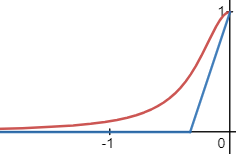float ChebyshevUpperBound(float2 moments,
float minVariance,
float lightBleedingReduction)
{
float variance = moments.y - (moments.x * moments.x);
variance = max(variance, minVariance); // 防止0除

float d = receiverDepth - moments.x;
float p_max = variance / (variance + d * d);

p_max = ReduceLightBleeding(p_max, lightBleedingReduction);

// 单边切比雪夫
return (receiverDepth <= moments.x ? 1.0f : p_max);
}


## 使用梯度对级联阴影采样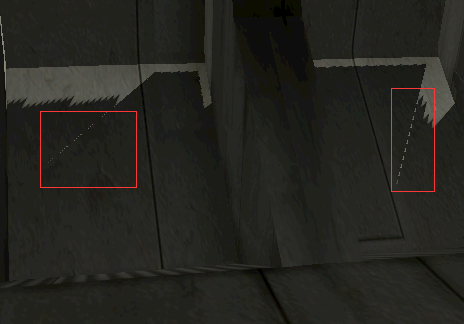float CalculateVarianceShadow(float4 shadowTexCoord,
{
float percentLit = 0.0f;

float2 moments = 0.0f;

// 为了将求导从动态流控制中拉出来，我们计算观察空间坐标的偏导
// 从而得到投影纹理空间坐标的偏导

percentLit = ChebyshevUpperBound(moments, shadowTexCoord.z, 0.00001f, g_LightBleedingReduction);

return percentLit;
}


## 优缺点总结

VSM具有如下优点：

• 可以使用图片空间blur或硬件filtering来产生软阴影

• 需要原来深度图占用显存空间的两倍来存放$d_o$$d_o^2$
• 在具有高方差分布的区域容易产生漏光（Light Bleeding）
• 大卷积核滤波会使漏光现象更加严重（因为方差值变大了）

$f(z)=e^{c(d-z)}, d<z, c>0f(z)=e^{c(d-z)}, d<z, c>0$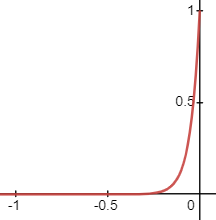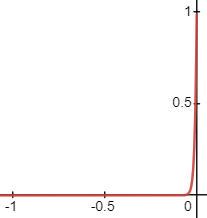## 提升精度

\begin{aligned}\sum_{i=0}^N w_i e^{cd_{o_i}}&= e^{cd_{o_0}}(w_0+\sum_{i=1}^Nw_i e^{c(d_{o_i}-d_{o_0})})\\ &=e^{cd_{o_0}}\cdot e^{ln(w_0+\sum_{i=1}^Nw_i e^{c(d_{o_i}-d_{o_0})})}\\ &=e^{cd_{o_0} + ln(w_0+\sum_{i=1}^Nw_i e^{c(d_{o_i}-d_{o_0})})} \end{aligned}

$cd_{o_0} + ln(w_0+\sum_{i=1}^Nw_i e^{c(d_{o_i}-d_{o_0})})$

## HLSL代码

float ESMLogGaussianBlurPS(float4 posH : SV_Position,
float2 texcoord : TEXCOORD) : SV_Target
{
float sum = g_BlurWeights[FLOAT_BLUR_KERNEL_SIZE / 2] * g_BlurWeights[FLOAT_BLUR_KERNEL_SIZE / 2];
[unroll]
for (int i = BLUR_KERNEL_BEGIN; i < BLUR_KERNEL_END; ++i)
{
for (int j = BLUR_KERNEL_BEGIN; j < BLUR_KERNEL_END; ++j)
{
float cdk = g_TextureShadow.Sample(g_SamplerPointClamp, texcoord, int2(i, j)) * (float) (i != 0 || j != 0);
sum += g_BlurWeights[i - BLUR_KERNEL_BEGIN] * g_BlurWeights[j - BLUR_KERNEL_BEGIN] * exp(cdk - cd0);
}
}
sum = log(sum) + cd0;
sum = isinf(sum) ? 84.0f : sum;  // 防止溢出
return sum;
}

//--------------------------------------------------------------------------------------
// ESM：采样深度图并返回着色百分比
//--------------------------------------------------------------------------------------
{
float percentLit = 0.0f;

float occluder = 0.0f;

percentLit = saturate(exp(occluder - g_MagicPower * shadowTexCoord.z));

return percentLit;
}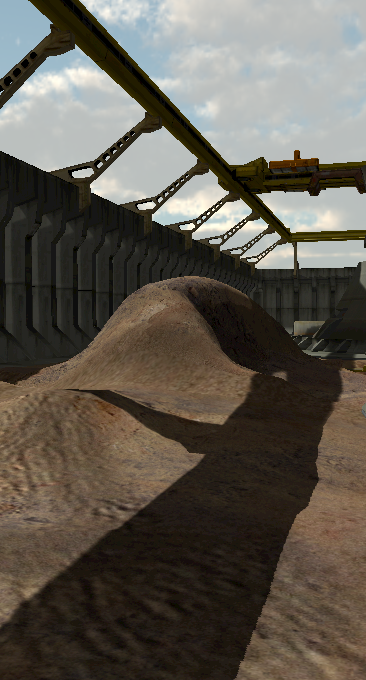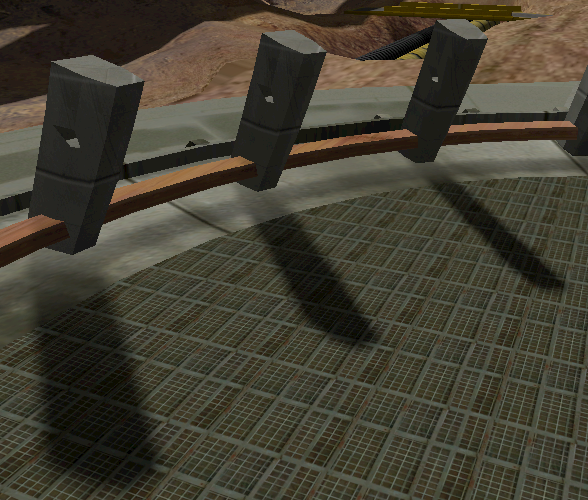## 优缺点总结

ESM具有如下优点：

• 可以使用图片空间blur或硬件filtering来产生软阴影，也不需要开很大的Blur
• 相比VSM只需要用1个float

• 为了提升精度需要用特定的Blur，在blur的过程会牺牲一定效率
• 邻近像素深度变化较大或位于非平面区域的话blur可能会失效
• 近处漏光问题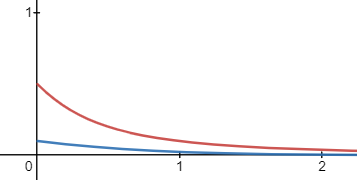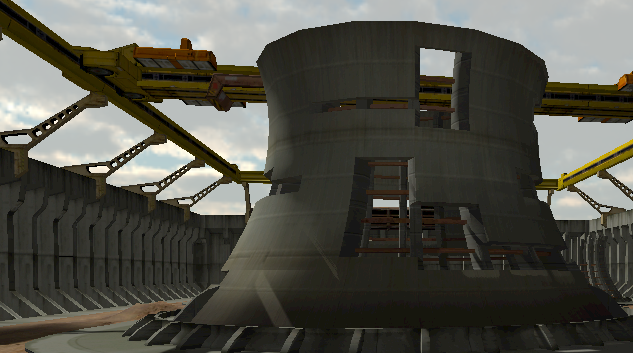## HLSL代码

EVSM的HLSL代码如下：

float2 GetEVSMExponents(in float positiveExponent, in float negativeExponent, in int is16BitFormat)
{
const float maxExponent = (is16BitFormat ? 5.54f : 42.0f);

float2 exponents = float2(positiveExponent, negativeExponent);

// 限制指数范围防止出现溢出
return min(exponents, maxExponent);
}

// 输入的depth需要在[0, 1]的范围
float2 ApplyEvsmExponents(float depth, float2 exponents)
{
depth = 2.0f * depth - 1.0f;
float2 expDepth;
expDepth.x = exp(exponents.x * depth);
expDepth.y = -exp(-exponents.y * depth);
return expDepth;
}

float2 EVSM2CompPS(float4 posH : SV_Position,
float2 texCoord : TEXCOORD) : SV_Target
{
uint2 coords = uint2(posH.xy);
float2 exponents = GetEVSMExponents(g_EvsmExponents.x, g_EvsmExponents.y, g_16BitShadow);
float2 outDepth = float2(depth.x, depth.x * depth.x);
return outDepth;
}

float4 EVSM4CompPS(float4 posH : SV_Position,
float2 texCoord : TEXCOORD) : SV_Target
{
uint2 coords = uint2(posH.xy);
float4 outDepth = float4(depth, depth * depth).xzyw;
return outDepth;
}

float CalculateExponentialVarianceShadow(float4 shadowTexCoord,
{
float percentLit = 0.0f;

float2 exponents = GetEVSMExponents(g_EvsmPosExp, g_EvsmNegExp, g_16BitShadow);
float4 moments = 0.0f;

percentLit = ChebyshevUpperBound(moments.xy, expDepth.x, 0.00001f, g_LightBleedingReduction);
if (SHADOW_TYPE == 4) // EVSM4
{
float neg = ChebyshevUpperBound(moments.zw, expDepth.y, 0.00001f, g_LightBleedingReduction);
percentLit = min(percentLit, neg);
}

return percentLit;
}## 优缺点总结

EVSM具有如下优点：

• 可以使用图片空间blur或硬件filtering来产生软阴影，也不需要开很大的Blur
• 相比前面的ESM、VSM，最大程度上减缓漏光问题
• 不需要使用很大的c，因此可以考虑使用16位float存储EVSM

• EVSM4需要使用4个float，不仅占用了一定的带宽，而且进行混合需要消耗更多的时间，对移动端可能不友好
• 极端情况下，存在ESM和VSM同时无法解决的artifacts

# 后记

• Light Bleeding：将[0, amount]映射到0，将[amount, 1]映射到[0, 1]
• Enable Mipmap：级联阴影开启mipmap
• Sampler：采样VSM使用的滤波
• Blur Sigma：高斯滤波用于控制权重分散情况
• Magic Power：控制$e^{cd}$$e^{cz}$的c项
• Pos Exp：控制$c_{pos}$
• Neg Exp：控制$c_{neg}$

# 参考与扩展阅读材料

## Fixed-Size Penumbra

• PCF(Percentage Closer Filtering)

## Variable-Size Penumbra

• VSSM = PCSS + VSM(Variance Soft Shadow Maps)
• SAVSM = VSM + SAT(Summed Area Table)

## Others

• 距离场阴影
• 光线追踪白给的阴影，但需要显卡支持

• GPU-Driven Cascade Setup and Scene Submission